Question

# Let ?, ?, and ? be independent random variables, uniformly distributed over [0,5], [0,1], and [0,2]...

Let ?, ?, and ? be independent random variables, uniformly distributed over [0,5], [0,1], and [0,2] respectively. What is the probability that both roots of the equation ??^2+??+?=0 are real?

Given that:

Let A, B, and C be independent random variables, uniformly distributed over [0,5], [0,15], and [0,2] respectively.

Ax2+Bx+C=0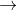For real solution

B2 - 4 AC > 0

B2 > 4 AC

Since

A = [ 0,5]

B = [0,1]

C = [0,2]

B2 > 4 AC

so we can square root both side

B> 2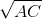Also , maximum value of 2= 2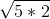= 2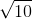Which is less than maximum value of B = 1

Limits

0 < A < 5

0 < C < 2

2< B < 1

Volume over which we are integrating:

5*2*1 = 10

So you must divide by 10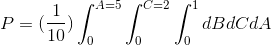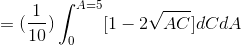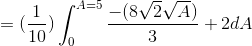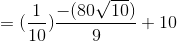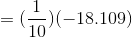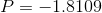#### Earn Coins

Coins can be redeemed for fabulous gifts.

Similar Homework Help Questions
• ### (1 point) Let A, B, and C be independent random variables, uniformly distributed over [0,4], [O,7],...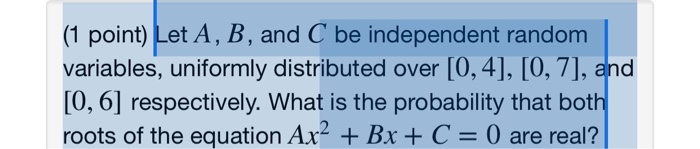(1 point) Let A, B, and C be independent random variables, uniformly distributed over [0,4], [O,7], and [0, 6] respectively. What is the probability that both roots of the equation Ax2 Bx+ C = 0 are real? (1 point) Let A, B, and C be independent random variables, uniformly distributed over [0,4], [O,7], and [0, 6] respectively. What is the probability that both roots of the equation Ax2 Bx+ C = 0 are real?

• ### Let A, B, and C be independent random variables, uniformly distributed over [0,6], [0,7], and [0,11]...

Let A, B, and C be independent random variables, uniformly distributed over [0,6], [0,7], and [0,11] respectively. What is the probability that both roots of the equation Ax2+Bx+C=0 are real?

• ### Let Y_(1) and Y_(2) be independent and uniformly distributed random variables over the interval (0,1). Find...

Let Y_(1) and Y_(2) be independent and uniformly distributed random variables over the interval (0,1). Find P(2 Y_(1)<Y_(2)).

• ### If Y is uniformly distributed over (0,5), what is the probability that both roots of 3x2...

If Y is uniformly distributed over (0,5), what is the probability that both roots of 3x2 + 3xY +Y +2=0 are real?

• ### Let X, Y be iid random variables that are both uniformly distributed over the interval (0,1)....

Let X, Y be iid random variables that are both uniformly distributed over the interval (0,1). Let U = X/Y. Calculate both the CDF and the pdf of U, and draw graphs of both functions.

• ### Let X1, Y.X2, ½, distributed in [0,1], and let ,X16, Y16 be independent random variables, uniformly...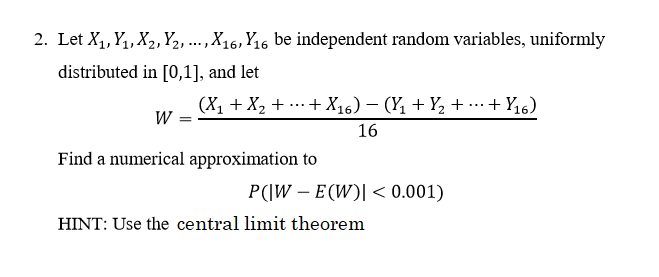Let X1, Y.X2, ½, distributed in [0,1], and let ,X16, Y16 be independent random variables, uniformly 2. 16 Find a numerical approximation to P(IW E(W)l< 0.001) HINT: Use the central limit theorem

• ### 1. Let U be a random variable that is uniformly distributed on the interval (0,1) (a)...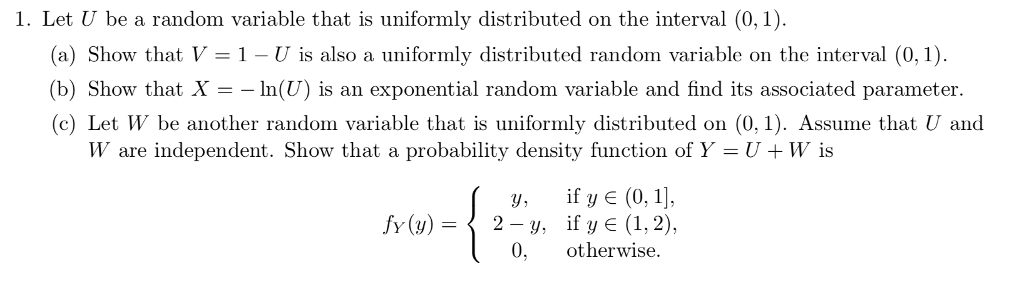1. Let U be a random variable that is uniformly distributed on the interval (0,1) (a) Show that V 1 - U is also a uniformly distributed random variable on the interval (0,1) (b) Show that X-In(U) is an exponential random variable and find its associated parameter (c) Let W be another random variable that is uformly distributed on (0,1). Assume that U and W are independent. Show that a probability density function of Y-U+W is y, if y E...

• ### Рroblem 5. Let X1. X2,.. be independent random variables that are uniformly distributed over [-1.1. Show...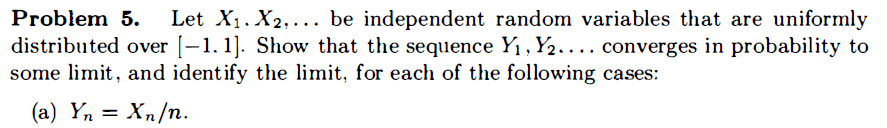Рroblem 5. Let X1. X2,.. be independent random variables that are uniformly distributed over [-1.1. Show that the sequence Yı , V2.... converges in probability to some limit, and ident ify the limit, for each of the following cases: (а) Ү, Хn/п. n

• ### 1 point) If a is uniformly distributed over [−27,23], what is the probability that the roots...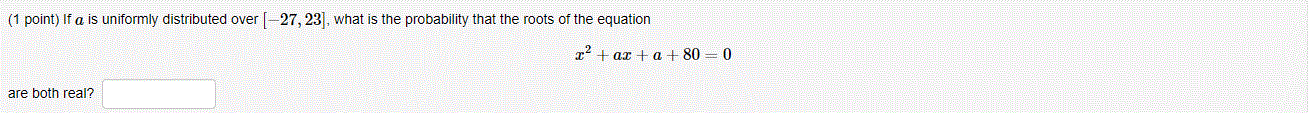1 point) If a is uniformly distributed over [−27,23], what is the probability that the roots of the equation x2+ax+a+80=0 are both real? (1 point) If a is uniformly distributed over [-27, 23), what is the probability that the roots of the equation r+ ax + a + 80 = 0 are both real?

• ### Let X and Y be independent random variables uniformly distributed on the interval [1,2]. What is ...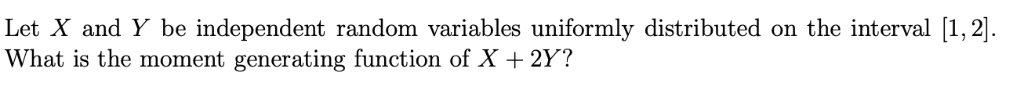Let X and Y be independent random variables uniformly distributed on the interval [1,2]. What is the moment generating function of X + 2Y? Let X and Y be independent random variables uniformly distributed on the interval [1,2]. What is the moment generating function of X + 2Y?